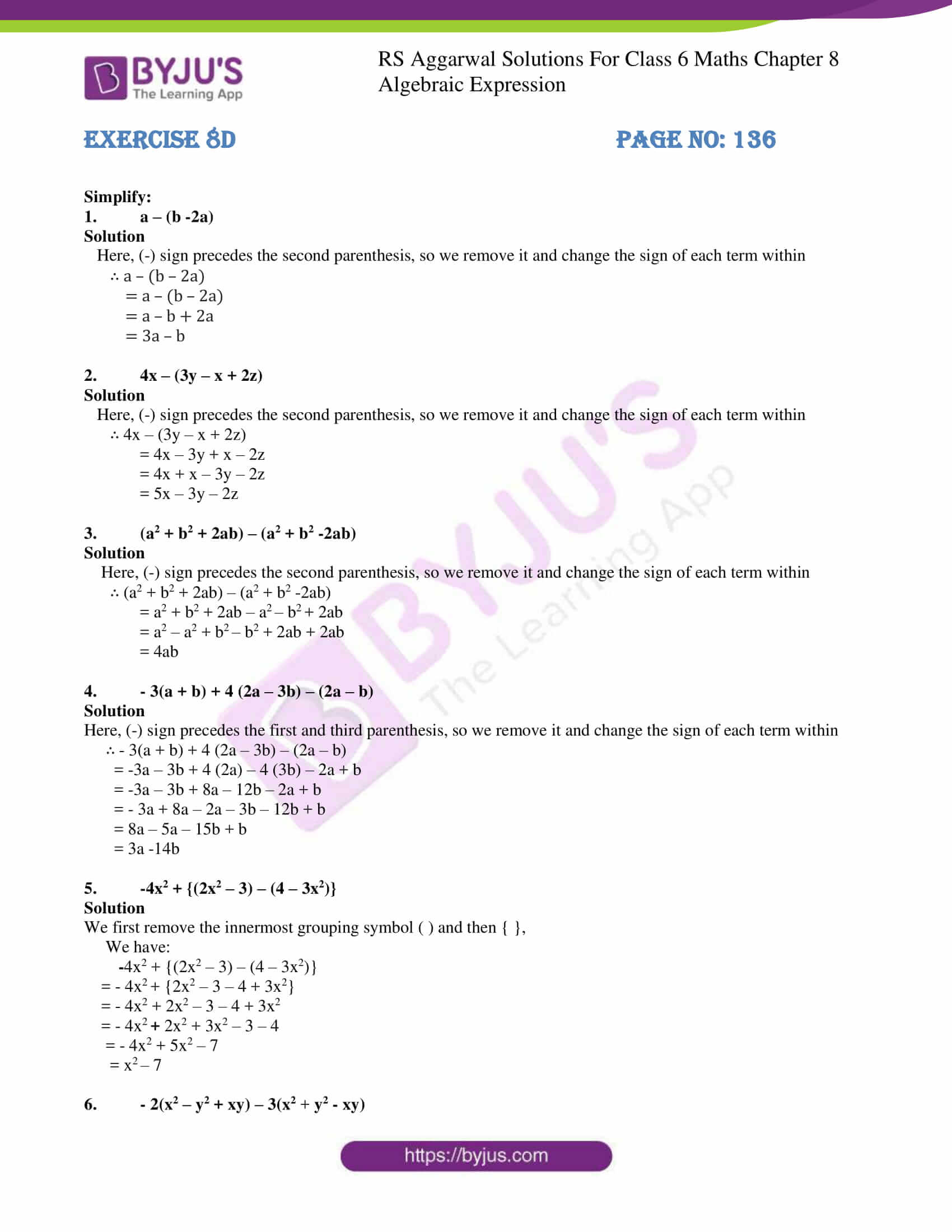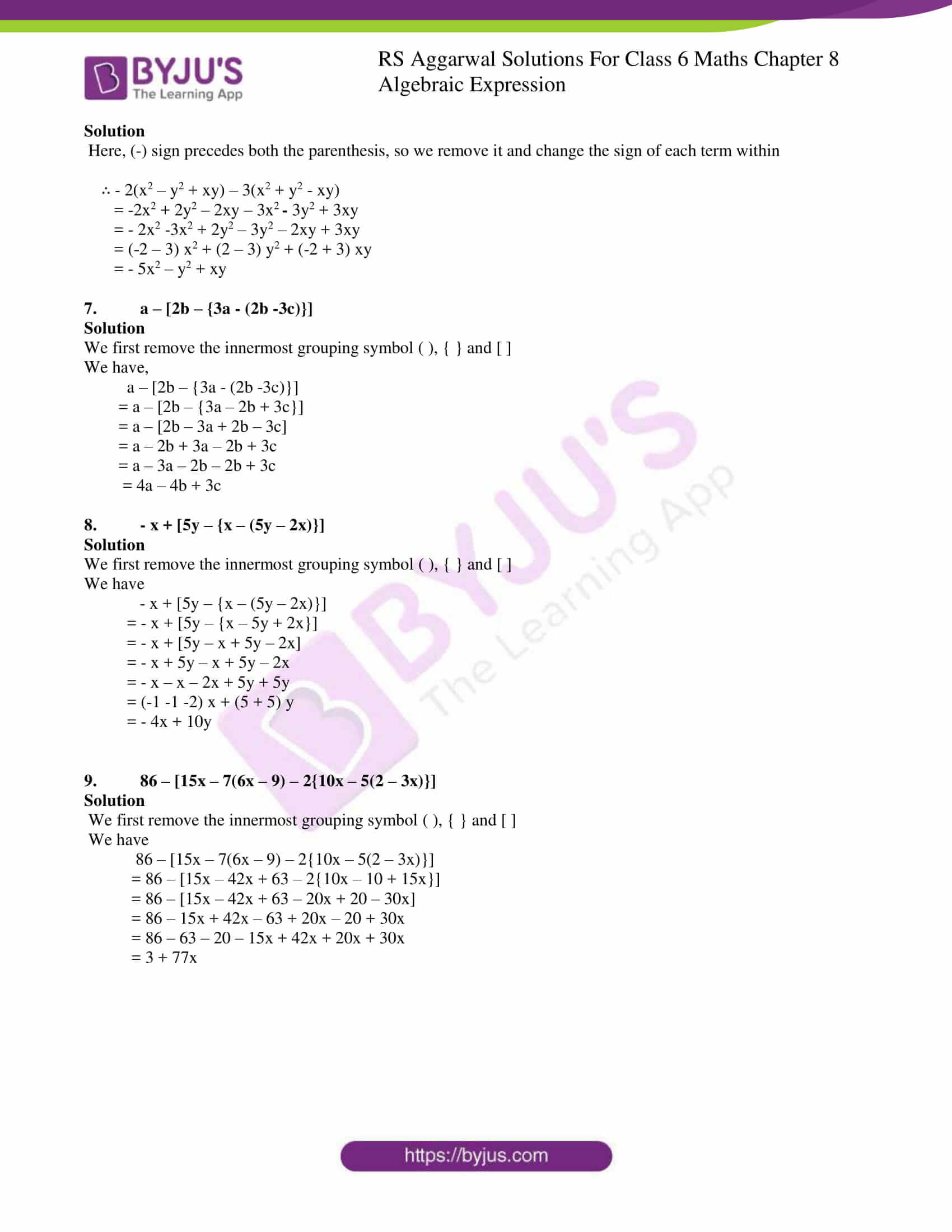# RS Aggarwal Solutions for Class 6 Chapter 8 Algebraic Expressions Exercise 8D

RS Aggarwal Solutions for Class 6 Chapter 8 Algebraic Expressions Exercise 8D are given here in PDF. Exercise 8D has the questions based on the topic use of grouping symbols. When we make operations on two or more Algebraic Expressions, we separate them by the symbols of groupings, namely parenthesis, braces and brackets. Students are advised to go through RS Aggarwal Solutions for better learning as they provide accurate answers. Practising RS Aggarwal Solutions help the students to solve even the toughest problems with ease. Download PDF from their respective links.

## Download PDF of RS Aggarwal Solutions for Class 6 Chapter 8 Algebraic Expressions Exercise 8D### Access answers to Maths RS Aggarwal Solutions for Class 6 Chapter 8 Algebraic Expressions Exercise 8D

Simplify:

1. a – (b -2a)

Solution

Here, (-) sign precedes the second parenthesis, so we remove it and change the sign of each term within

∴ a – (b – 2a)

= a – (b – 2a)

= a – b + 2a

= 3a – b

2. 4x – (3y – x + 2z)

Solution

Here, (-) sign precedes the second parenthesis, so we remove it and change the sign of each term within

∴ 4x – (3y – x + 2z)

= 4x – 3y + x – 2z

= 4x + x – 3y – 2z

= 5x – 3y – 2z

3. (a2 + b2 + 2ab) – (a2 + b2 -2ab)

Solution

Here, (-) sign precedes the second parenthesis, so we remove it and change the sign of each term within

∴ (a2 + b2 + 2ab) – (a2 + b2 -2ab)

= a2 + b2 + 2ab – a2 – b2 + 2ab

= a2 – a2 + b2 – b2 + 2ab + 2ab

= 4ab

4. – 3(a + b) + 4 (2a – 3b) – (2a – b)

Solution

Here, (-) sign precedes the first and third parenthesis, so we remove it and change the sign of each term within

∴ – 3(a + b) + 4 (2a – 3b) – (2a – b)

= -3a – 3b + 4 (2a) – 4 (3b) – 2a + b

= -3a – 3b + 8a – 12b – 2a + b

= – 3a + 8a – 2a – 3b – 12b + b

= 8a – 5a – 15b + b

= 3a -14b

5. -4x2 + {(2x2 – 3) – (4 – 3x2)}

Solution

We first remove the innermost grouping symbol ( ) and then { },

We have:

4x2 + {(2x2 – 3) – (4 – 3x2)}

= – 4x2 + {2x2 – 3 – 4 + 3x2}

= – 4x2 + 2x2 – 3 – 4 + 3x2

= – 4x2 + 2x2 + 3x2 – 3 – 4

= – 4x2 + 5x2 – 7

= x2 – 7

6. – 2(x2 – y2 + xy) – 3(x2 + y2 – xy)

Solution

Here, (-) sign precedes both the parenthesis, so we remove it and change the sign of each term within

∴ – 2(x2 – y2 + xy) – 3(x2 + y2 – xy)

= -2x2 + 2y2 – 2xy – 3x2 3y2 + 3xy

= – 2x2 -3x2 + 2y2 – 3y2 – 2xy + 3xy

= (-2 – 3) x2 + (2 – 3) y2 + (-2 + 3) xy

= – 5x2 – y2 + xy

7. a – [2b – {3a – (2b -3c)}]

Solution

We first remove the innermost grouping symbol ( ), { } and [ ]

We have,

a – [2b – {3a – (2b -3c)}]

= a – [2b – {3a – 2b + 3c}]

= a – [2b – 3a + 2b – 3c]

= a – 2b + 3a – 2b + 3c

= a – 3a – 2b – 2b + 3c

= 4a – 4b + 3c

8. – x + [5y – {x – (5y – 2x)}]

Solution

We first remove the innermost grouping symbol ( ), { } and [ ]

We have

– x + [5y – {x – (5y – 2x)}]

= – x + [5y – {x – 5y + 2x}]

= – x + [5y – x + 5y – 2x]

= – x + 5y – x + 5y – 2x

= – x – x – 2x + 5y + 5y

= (-1 -1 -2) x + (5 + 5) y

= – 4x + 10y

9. 86 – [15x – 7(6x – 9) – 2{10x – 5(2 – 3x)}]

Solution

We first remove the innermost grouping symbol ( ), { } and [ ]

We have

86 – [15x – 7(6x – 9) – 2{10x – 5(2 – 3x)}]

= 86 – [15x – 42x + 63 – 2{10x – 10 + 15x}]

= 86 – [15x – 42x + 63 – 20x + 20 – 30x]

= 86 – 15x + 42x – 63 + 20x – 20 + 30x

= 86 – 63 – 20 – 15x + 42x + 20x + 30x

= 3 + 77x